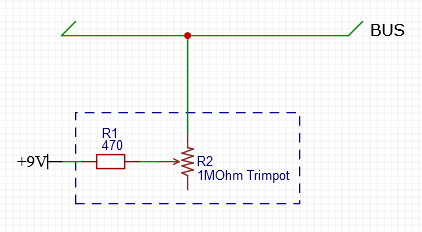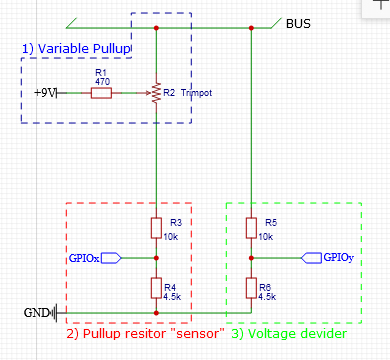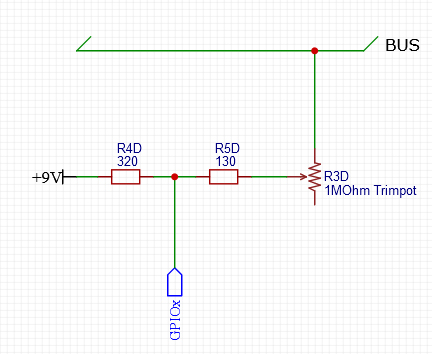# Howto measure BUS rise time + variable pullup resistor

Hi all.

I previously posted this followup question in another post, but I guess the question was too off topic

I would like to measure the rise time of a BUS communication cable which could be I2C, SPI or any other communication type. In my case I will be using I2C with 9V buffer, but in principal this question applies to any other communication type as well.

In principal, I will be using a resistor with trimpot R2 in series to have an adjustable pullup resistor (See image 1 below). R1 is the the minimum resistance required when using the shortest communication cable, it also serves as a safeguard to prevent a short circuit. R2 is the adjustable trimpot rated to the maximum resistance calculated for the longest cable situation.

Image 1My communication cable can vary considerably, I would like to create a method to measure the rise time using an Arduino to then setup the right value of the trim pot, optionally even read the trimpot value too. However, I do not want the measurements to pulldown the bus or negatively influence the readings.

Image 2 shows my initial flawed thought, but I think that setup would react like a pulldown resistor on the BUS, or not?

Image 2.. Initial ideaThe idea was to add a voltage divider at 2) between trimpot & ground to measure the voltage at the trimpot, thus being able to calculating the total pulldown resistor value. But, the voltage divider would also act as a pulldown resistor?

A second voltage divider 3) between bus and GND would measure the voltage rise over time... again this would act like a pulldown resistor. In general, reading the voltage is preferred over just High/Low readings (the high/low values of components can differ)

Even adding a transistor to isolate the voltage dividers after setup would not really help, as the readings during setup are influenced. I also was contemplating measuring the voltage difference between bus and +9V instead of ground, but again, it would be like adding a resistor in parallel to the pullup resistor.

So my question is, how does one measure rise time with an Arduino without negatively influencing a BUS reading?

Mike

You will never want a reasonable resistor between a bus and connected outputs because it will not only slow down the rise and fall times but also will reduce the signal swing (s/n ratio).

Available bus extenders add active pull up/down transistors that speed up a detected level change until a safe level is reached.

Thanks Diettrich.

Oscilloscope is a nice thing to have, question is then would it be worth it. Obviously a oscilloscope also influences the signals to a degree. I did have a look at some input circuit diagrams from oscilliscopes now, they tend to use high Ohm resistors (10MOhm) and some capacitors.

Other thought I had was, measuring the potential differential between BUS and +9V to calculate the rise time (Image3)... but the bus side would be ground then and adding an additional 10MOhm resistor would mean I need amplification... and then it becomes complicated...

Image3I guess your right... get an oscilloscope and rather provide easy accessible connectors to the PCB for the probe. The added costs for 50 PCBs would also cost as much as an oscilloscope but be less flexible.

1. Avoid the voltage divider entirely, use the microcontroller's Vcc as the test voltage. Simpler, better. I'd also worry that 9V might damage devices on the bus. If all the devices are not connected to the bus, their input capacitance is not being measured, and this might be a source of significant error.

2. You are measuring the capacitance of the bus, and there are several ways to do this, specifically you don't need a variable resistor to do this - its a complication that's not needed (its also a problem in that you have to measure its value somehow).

You might use charge injection and measure the voltage step produced - this can be done with a fixed resistor (or a small set of selectable fixed resistors) and variable pulse time - the pulse time is something the code has control of and won't need to measure separately. The simple equation is delta-V = Q/C, where Q is the charge injected

This requires to capture the peak voltage - another extension of the interface. The Arduino CapSense library uses the opposite way, injects a current until a certain voltage level (threshold) is reached.

SPI typically works at controller clock, no chance to measure a real rise time smaller than a clock pulse.

I2C rise time depends on the pullup resistors on the wires. Meaningful measurement is possible only in the field, with all modules installed and wired up. Or you have to install an equivalent pullup resistor on a properly sized cable.

No, just measure the change in voltage before and after injecting charge - capacitors integrate charge. It does require leakage currents to be small enough not to matter on the timescale of the two measurements though. But its easier to adjust a pulse width to fractions of a microsecond than take analog measurements a fraction of a microsecond apart.

I think the point of this question is to determine what resistor value is needed for a given cable and risetime requirement, ie measure the capacitance.

Personally I'd just calculate the capacitance from the datasheet for the cable, its length, and the input capacitances of the loads, then multiply by a suitable safety factor. Twisted pair is around 50pF/m, most coax is around 80pF/m.

But note that fast logic busses like I2C and SPI are not designed to travel far, so really there shouldn't be much cable anyway. Long cables bring problems like ringing and reflections so you need to worry about those too.

This would mean one circuit to inject charge and another one to unload the capacity.

A digital pin with a resistor, and analog pin to sense voltage - that's a one resistor circuit!
Digital pins can be switched to INPUT mode which it high impedance, you'd do that before and after charge injection.

This topic was automatically closed 120 days after the last reply. New replies are no longer allowed.